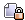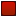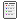###Author Topic: Vol Compression  (Read 966 times)

####Hooman

• Hero Member
••• Posts: 4871##### Vol Compression
« on: August 03, 2007, 12:06:11 AM »
There are 4 possible storage methods used in .vol files. The type of compression used is indicated by a byte in the index entry for that file. The release version of Outpost 2 uses only types 0 and 3. Type 3 was used for the .txt files, and everything else used type 0. The other types could however be used by any newly authored .vol files. The code to compress and decompress these type exists inside Outpost2.exe. The compression schemes are as follows.

Compression Type: 0  [Uncompressed]
The file contents are simply stored as is in the .vol file.

Compression Type: 1  [RLE]
The file is Run Length Encoded. The file is stored in small sections, where each section begins with a header byte. The most significant bit of the header byte is used to determine which type of block follows, and the remaining 7 lower bits are used as a count field. If the most significant bit was 0, then a group of bytes of length given by the count field is copied directly to the output. If the most significant bit was a 1, then the next byte is read, and repeated count times to the output. The file is then just a sequence of these sections, each starting with a header byte, and followed by either a block of bytes to simply output, or a single byte to repeat.

Compression Type: 2  [LZ]
The file is compressed using an LZ style compression algorithm, and can encode runs of previously seen bytes. The file is stored as a bit stream. Data elements are in general not byte aligned. The bit stream is ordered such that the most significant bit of each byte comes before the other bits of that byte in the stream. When a group of bits forms a binary value, the first bit in the stream is the most significant bit of that binary value. A 4096 byte circular buffer is used to encode/decode runs of previously seen bytes. Everytime a byte is encoded/decoded it is copied to the circular buffer. There are two types of codes used by this file type. A single lead bit is used to determine which code type follows. If the lead bit is a 1, then the code type is a single raw output byte. This byte is the next 8 bits following the lead bit. If this lead bit is a 0, then a repeated run type of code follows. The repeated run code consists of a 12 bit (absolute) index into the circular buffer of where the repeated run starts, and then a 4 bit run length. The actual run length is the binary values of those 4 bits + 1. Hence, the 12 bit index can index to any position in the 4096 byte circular buffer, and the run length can be from 1 to 16 bytes. The file is then just a sequence of lead bits followed by the appropriate code for the raw byte or the encoded run.

When decoding it is important to update the circular buffer after each byte output, so that it always contains the most recent 4096 bytes (provided at least that many bytes have been output to/from the stream). This is particularly improtant since a run may start directly behind the write index, so that as each byte is read from the circular buffer and output, it is also copied back into the circular buffer at the current write index. That way when the next byte in the run is read from the circular buffer, it sees the new value that was just written. The circular buffer is not initialized before decoding.

Compression Type: 3  [LZH]
The file is compressed using adaptive Huffman codes, and can encode runs of previously seen bytes. The file is stored as a bit stream. Data elements are in general not byte aligned. The bit stream is ordered such that the most significant bit of each byte comes before the other bits of that byte in the stream. When a group of bits forms a binary value, the first bit in the stream is the most significant bit of that binary value. A 4096 byte circular buffer is used to encode/decode runs of previously seen bytes. Of this 4096 byte buffer, 4036 consecutive bytes of the circular buffer are initialized to 32 (0x20 = " "). The remaining 60 bytes of the circular buffer are left uninitialized. The write index points to the first uninitialized byte when encoding/decoding begins. There are 314 codes, of which 256 represent corresponding raw bytes, and 58 represent repeated runs. The length of the run is given by adjusting the codes for the repeated runs, so that runs of length 3 to 60 bytes are possible. The offset within the circular buffer of where the run starts is placed in the bit stream following the bits representing the length of the run. The offset of the run within the circular buffer is given relative to the current write index minus one, and represents how many bytes behind the write index the run starts at. Thus an offset of 0, means the run starts 1 byte behind the write index, and an offset of 1, would mean the run starts 2 bytes behind the write index. Details on how to read the offset bits from the bit stream will be given later.

When decoding it is important to update the circular buffer after each byte output, so that it always contains the most recent 4096 bytes (provided at least that many bytes have been output to/from the stream). This is particularly improtant since a run may start directly behind the write index, so that as each byte is read from the circular buffer and output, it is also copied back into the circular buffer at the current write index. That way when the next byte in the run is read from the circular buffer, it sees the new value that was just written.

There are two types of bit groups in the compressed stream. The first are the Huffman codes, which repesent the 314 codes discussed above. The other bit groups represent offsets of runs. These are treated differently from the Huffman codes.

Note: The term Huffman code will be used to denote what is found in the compressed stream, and the term code will be used to denote the intermediate 314 values that represent either a raw character or a run length. The bit representation of the codes in the compressed stream (i.e. the Huffman codes) change throughout the stream. That is, they are adaptive Huffman codes. They change in such a way that the more frequent codes get progressively shorter Huffman codes associated with them, and thus The less frequently used codes will, over time, be associated with longer Huffman codes.

An adaptive Huffman tree can be used to maintain the mapping between the codes representing the raw bytes or run lengths with the actual Huffman codes stored in the file. An appropriate adaptive Huffman tree that initially represents the correct mapping between codes and Huffman codes can be constructed by creating a left justified balanced binary tree with 314 terminal nodes, where the codes stored in the leafs increase from left to right from 0 to 313, with codes 0-255 representing raw bytes of that same value, and codes 256-313 represent runs of length (code - 253). Note that a left justified balanced binary tree with 314 terminal nodes is a tree with 116 terminal nodes at distance 9 from the root along the left of the tree, and 198 terminal nodes at distance 8 from the root along the right part of the tree. That is, the extra weight is shifted to the left of the tree, since not every node in a tree this size can be the same distance from the root. (Balanced being all terminal nodes are at maxDepth, or maxDepth - 1, and left justified being which side of the tree to stick the deeper nodes on). This means codes 0 to 117 will have a 9 bit Huffman code representation initially, and codes 118 to 313 will have an 8 bit Huffman code representation initially. The Huffman code is then the path from the root to the leaf, where a 0 bit means the left link of the parent, and a 1 bit means the right link of a parent. The first bit of the Huffman code (appearing first in the bit stream) is the bit corresponding to the link used between the root node and it's child, and the last bit in the Huffman code is the bit corresponding to the link between the parent of the leaf and the leaf. The frequency count of all terminal/leaf nodes should be initialized to 1, and the frequency count of each internal/parent node is the sum of the frequencies of it's two children. Tree update follows the standard algorithm. When the frequency count for a leaf is updated, the change is propagated upwards to the root, incrementing the frequency count at each node along the path from the leaf to the root, but performing tree restructuring along the way, so that the final path of increments from the leaf to the root was not the original path from the leaf to the root. At each step the current node is swapped with it's block leader. The block leader is the highest up and rightmost node with the same frequency count as the current node, before the frequency count for the current node is updated. After every code is encoded/decoded using the tree, the tree must then be restructured according to the previous frequency count propogation algorithm to represent the new mapping between codes and Huffman codes. It is important to restructure after encoding/decoding a code, so that the compressor and decompressor can stay in sync.

The offset of a run within the circular buffer is stored as a block of 9 to 14 bits following the Huffman code for the length of the repeated run. The offset bits are not encoded/decoded using the adaptive Huffman codes. Thus there is no tree lookup/update pair when reading/writing these blocks of bits. They are however still Huffman codes. (Canonical Huffman codes actually). Although the entire 9 to 14 bit codes can be considered Huffman codes, it's easier to break them up into two parts. Consider the first 3 to 8 bits to be a Huffman code, and the following 6 bits as a fixed block of bits to be taken as is. This is like breaking up the 12 bit index into the 4096 byte circular buffer into a high 6 bits and a low 6 bits. The high 6 bits correspond to the 3 to 8 bit Huffman code, and the 6 low bits correspond to the later 6 bits following the 3 to 8 bit Huffman code. This interpretation allows for a simpler description of the translation between the codes and their corresponding Huffman codes, and if a table is used to translate between the two forms, it makes the table smaller. Note that since these Huffman codes are fixed, encoding and decoding can be done faster using table lookups, processing a block of bits at once, rather than with a tree, processing 1 bit at a time. The table can be precomputed at compile or design time. (Note that table updates are significantly slower than tree updates, so this table method of encoding/decoding doesn't apply very well to adaptive Huffman codes).

This encoding/decoding table can be generated quite easily using a standard Canonical Huffman code algorithm. Generally the length of each symbol (code) being encoded to a codeword (to a Huffman code) is enough to generate the table. The symbols are usually sorted first on the length of the codeword (Huffman code) as the primary sorting key, and then within each block the symbols are sorted lexicographically. In our case, the symbols we wish the encode are the 6 high order bits, with values from 0 to 63. It also happens that the length of the codwords for each of these symbols is monotonically increasing, which basically means our sorted list is just the numbers 0 to 63 in that order. Thus for our purposes, we can skip the sorting step. We now assign codewords to these symbols as follows. Initialize a counter to 0, and keep track of the current code length. In our case, the code length starts at 3 and increases up to 8. We will assign the appropriate number of lower order bits of the counter as the codeword for the current symbol. After each symbol, we will increment the counter, and if the length of the codeword increases for the next symbol, we will also shift the counter left by 1, and append a 0 (that is, multiply the counter by 2). Note that the increment is done before the shift, and the assignment of the codeword to the symbol is done last. Here's a quick example:

Symbol  Code Length  Codeword
------  -----------  --------
0   3      000
1   4      0010
2   4      0011
3   4      0100

4   5      01010
5   5      01011
6   5      01100
7   5      01101

The complete table can be specified by giving the lengths of the codewords for each of the 64 symbols (codes), and following the algorithm for generating the table. Instead, since the symbols are already in order, where the code lengths are monotonically increasing, and the lengths always increase by 1 each time, starting from 3 and ending at 8, a simple list of the number of consecutive codewords of the same length will suffice. The table can then be specified as follows: [1, 3, 8, 12, 24, 16]. That is, one code of length 3, followed by 3 codes of length 4, then 8 codes of length 5, then 12 codes of length 6, then 24 codes of length 7, and finally 16 codes of length 8. The example above gives the first few codewords that would be generated in this setting. The leftmost bit in the example is the one that appears first in the stream. Examples of encoded offsets follow, where a "|" is placed between the Huffman code for the high order bits, and the 6 low order bits:

Offset  Huffman Code
------  ------------
0   000 | 000000
63   000 | 111111
64   0010 | 000000
193   0100 | 000001
500   01101 | 110100

Remember that the actual start of the run in the circular buffer is at (writeIndex - offset - 1), so an offset value of 0 means to start one byte behind the write pointer. With this form of encoding, it takes fewer bits to encode runs that are more recent, but can still encode runs that are as far back as 4096 bytes. Hence a fairly large region can be checked for runs, while not forcing a large number of bits to be used to encode the offsets of runs that are not far apart. The idea likely being that runs which are close together are more likely than runs which are far apart.

The compressed stream is then a sequence of adaptive Huffman codes, where after each Huffman code representing the length of a run, there are additional bits forming a fixed Huffman code representing the offset of the run.
« Last Edit: August 06, 2007, 12:26:28 AM by Eddy-B »